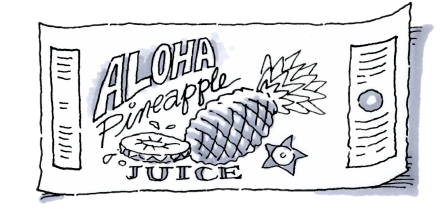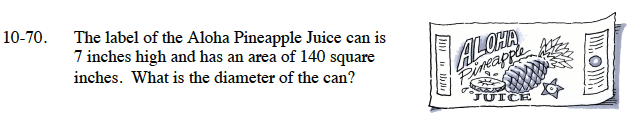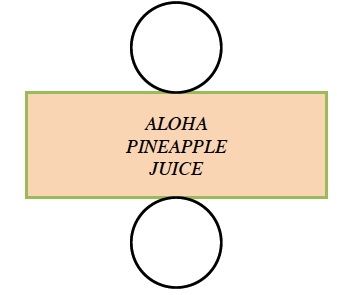Home > ACC7 > Chapter cc310 > Lesson cc310.1.5 > Problem10-70

10-70.The label of the Aloha Pineapple Juice can is 7 inches high and has an area of 140 square inches. What is the diameter of the can? Homework Help ✎The label wraps around a cylinder.
It may be useful to draw a net of this cylinder.You know the equation for the area of a rectangle can be found using the formula:
Area = (base)(height)
Substitute the known values and solve for the unknown value.

140 = (base)(7)

$\left(\frac{140}{7}\right)=\text{base}\left(\frac{7}{7}\right)$

base = 20 inches

You learned that the width of the rectangle is equal to the circumference of the circle. Substitute the known values into the following equation and solve for the diameter:
Circumference = (diameter)π

20 = (diameter) · π

$\frac{20}{\pi }=\left(\text{diameter}\right)\frac{\pi }{\pi }$

Diameter = ?